## Friday, 8 August 2014

### CHAPTER 9 - Rectangular Resolution

Consider two non-collinear vectors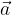$\vec a$ and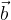$\vec b$; as discussed earlier, these will form a basis of the plane in which they lie. Any vector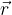$\vec r$ in the plane of$\vec a$ and$\vec b$can be expressed as a linear combination of$\vec a$ and$\vec b$The vectors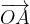$\overrightarrow {OA}$ and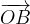$\overrightarrow {OB}$ are called the components of the vector$\vec r$ along the basis formed by$\vec a$ and$\vec b$. This is also stated by saying that the vector$\vec r$ when resolved along the basis formed by$\vec a$ and$\vec b$, gives the components$\overrightarrow {OA}$ and$\,\overrightarrow {OB}$. Also, as discussed earlier, the resolution of any vector along a given basis will be unique.
We can extend this to the three dimensional case: an arbitrary vector can be resolved along the basis formed by any three non-coplanar vectors, giving us three corresponding components. Refer to Fig –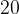$20$ for a visual picture.

#### RECTANGULAR RESOLUTION

Let us select as the basis for a plane, a pair of unit vector$\hat i$ and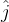$\hat j$ perpendicular to each other.Any vector$\vec r$ in this basis can be written as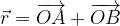$\vec r = \overrightarrow {OA} + \overrightarrow {OB}$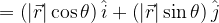$= \left( {\left| {\vec r} \right|\cos \theta } \right)\hat i + \left( {\left| {\vec r} \right|\sin \theta } \right)\hat j$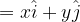$= x\hat i + y\hat j$
where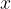$x$ and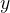$y$ are referred to as the$x$ and$y$ components of$\vec r$.
For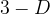$3-D$ space, we select three unit vectors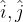$\hat i,\hat j$ and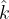$\hat k$ each perpendicular to the other two.In this case, any vector$\vec r$ will have three corresponding components, generally denoted by$x$$y$ and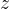$z$. We thus have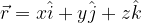$\vec r = x\hat i + y\hat j + z\hat k$
The basis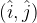$(\hat i,\hat j)$ for the two dimensional case and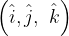$\left( {\hat i,\hat j,\,\,\hat k} \right)$ for the three-dimensional case are referred to as rectangular basis and are extremely convenient to work with. Unless otherwise stated, we’ll always be using a rectangular basis from now on. Also, we’ll always be implicitly assuming that we’re working in three dimensions since that automatically covers the two dimensional case.